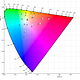# Computing 2D affine transformations using only matrix multiplication

This is a short visual description of computing a 2D affine transformation using a single matrix multiplication step, something that requires a bit of dimensional trickery. I’m trying to get better at check-pointing my math knowledge when I figure things out.

Say you have a bunch of points in two dimensional space (R2) and you want to translate (shift) them by 2 on the x axis.

You could formulate that with vector addition like so:

There’s a problem though, shifting all points to the right also means that your operation is going to shift the origin [0,0] to the right. This disqualifies this function from being a linear transformation.

Linear transformations have to preserve zero. They also can be expressed with a single matrix multiplication, no vector addition step needed. An example is the linear transformation for a rotation about the origin.

Combining a linear transformation like rotation with a translation step is a very common operation and it qualifies as a different type of transformation, an affine transformation.

An affine transformation has fewer rules, it no longer needs to preserve the origin it just has to keep straight lines straight and some other stuff

Affine operations like ‘rotate and translate’ are pretty useful and it would be helpful we could express them as a single matrix multiplication (Ax) instead of a multiplication and a vector addition (Ax + b).

It turns out that affine transformations in 2D can be represented as linear transformations in 3D. First let’s hoist our 2D space into 3D by making it a plane at z = 1.

Notice the old origin is now at (0,0,1) in our 3D space. This means we are free to map it to a value other than itself, as long as we preserve the 3D origin (0,0,0).

Here’s the recipe:

Since z is always 1 on our plane, b1 and b1 become constant additions, you can see this if you work it out a bit.

This lets us translate points on our plane by b amount

For any value of Z other than 1 (values not on our plane) b1 and b2 would end up scaled. We have effectively found a shearing of 3D space that happens to be our desired translation only at our plane. And if you work it out [0,0,0] is still mapped to [0,0,0] by this matrix.

You’re left with a translated point on your plane, now you can just drop the z component (which will be 1) and you’re back in your 2D space.

If you’re still trying to visualize how objects on the plane move but the 3D origin doesn’t just look up shears. In 2D you can see that a sheer leaves 0,0 alone.

Written by

Written by

## Chandler Abraham

#### Idaho not Iowa. Formerly @twitter, now I do space stuff. #RWEN